# On Energy Distribution in Cities: A Model Based on Complex Networks

On Energy Distribution in Cities: A Model Based on Complex Networks

Alberto Fichera Mattia Frasca Rosaria Volpe*

Dipartimento di Ingegneria Elettrica, Elettronica ed Informatica, viale Andrea Doria 6, 95125 Catania, Italy

Corresponding Author Email:
rvolpe@dii.unict.it
Page:
611-615
|
DOI:
https://doi.org/10.18280/ijht.340409
|
Accepted:
|
Published:
31 December 2016
| Citation

OPEN ACCESS

Abstract:

Rapid urbanization and the remarkable energy consumption deriving from urban areas require the definition of proper urban energy strategies for the achievement of energy efficiency. In the last year, Decentralized Energy Systems (DESs) have gained attention from the scientific community and from the local governments, since they allow the on-site production of energy, thus improving the efficiency of the energy systems by reducing energetic wastes and energy losses on transmission lines. Yet, the diffusion of DESs gives rise to energy interactions among users, defined as energy subjects with certain energy demand and with the chance to install autonomous energy generation systems. In order to consider the energy exchanges originating from those interactions, a mathematical procedure based on complex networks is strengthen. The elaborated method is then applied on a speculative territory in order to determine the optimal energy flows occurring between each node pair. Thus, the constructed model aims to be an advantageous tool for the proper definition of urban energy action plans focusing on the insertion of DESs on the urban territory.

Keywords:

Urban areas, Decentralized energy systems, Complex networks, Energy, Urban planning.

1. Introduction

Ongoing trends in rapid urbanization accentuate sustainability and energy efficiency challenges, in particular that of differentiate the energy supply [1, 2]. Moreover, being cities characterized by two-third of the global energy demand , the need to produce energy in a more responsive way is becoming even more urgent.

Traditionally, centralization is the common approach to the energy supply. Due to the centralized approach, energy is guaranteed by power plants that produce energy later distributed to final users. The alternate approach is decentralized; and, in this context, Decentralized Energy Systems (DESs) have been recognized as an effective option to centralization .

Despite the fact that cities are often seen as net consumers of energy, there are several opportunities for local energy generation in urban areas . In this direction, the scientific community offers various contributions to the installation of DESs in the urban area. Some authors focus on the insertion of solar energy systems in buildings [6 – 9]. In the meantime, others concentrate on the neighbor scale and present models for the optimal integration of DESs in urban areas [10, 11].

In addition, detailed studies deal with the choice of the optimal technology to invest in [12 – 14].

The integration of distributed generation in the urban territory entails that energy users will not be merely passive consumers, instead they switch between consumers and local energy producers. Moreover, energy flows, which are unidirectional in the traditional energy distribution system, turn into bidirectional flows.

The origin of bidirectional flows implicates that users, in the role of consumers or otherwise producers, establish energy interactions for which an in-depth analysis is suitable . In the matter in question, the decentralization of the energy generation also impacts on the urban planning and, therefore, pushes towards the definition of proper energy planning models centered on the installation of energy efficient systems in urban areas. The presented work proceeds in this direction by applying the framework of complex networks to the urban energy distribution, in order to analyze the energy interactions occurring among users. Complex networks are a widely treated topic in literature since they allow studying a system and the relations among its constituents . Furthermore, due to the high flexibility of applications that ranges from sociology to informatics, complex networks seem suitable to be also introduced in the urban energy distribution problem [17, 18]. In particular, nodes and links, the main elements of complex networks, permit the matching to the energy problem by identifying nodes as users and links as energy interactions. Specifically, users may generally be identified as dwellings, buildings, neighborhood, depending on the detail of the study. The association of urban areas as complex networks permits to identify as object of the study the hereinafter called urban energy network.

Hence, in this paper, a mathematical procedure for the optimization of the energy flows deriving from the installation of Decentralized Energy Systems in urban areas is developed and its application is discussed.

2. The Mathematical Model

The application of the complex network theory to the urban energy distribution problem results in a mathematical model that evaluates the energy interactions among users. To get into the details, a user is basically an energy subject that consumes energy. At the same time, the user may behave as producer due to the installation of an energy generation system. Therefore, a user is characterized by a certain energy demand and an energy generation. In particular, users employ the eventual generated energy primarily for the satisfaction of their own energy demand, and, subsequently, for the distribution of any exceeding energy.

As already introduced, nodes and links are the main constituents of network. Under the complex network framework, the urban energy network may be described as a collection of nodes and links, where nodes represent users and links the connections for the energy exchange.

To model an urban energy network, N nodes are distributed on a bi-dimensional space. Each node i, for i=1,…, N, is described by an energy demand Di and a potential energy generation Gi. Links are assigned on the basis of a vicinity criterion, for which the energy exchange between a nodes pair is feasible if their reciprocal distance d is within a given threshold. This hypothesis derives from the Italian Regulation of Efficient Systems for Users .

Concurrently with the potential connections with neighbors, each node gains a further connection with the power station, called central node and specifically distinguished from the other nodes of the set N. Thus, the initial set of nodes is enlarged to N+1, and, precisely, the central node is labeled as i=1.

All energy connections are described in a (N+1) x (N+1) matrix, called adjacency matrix. Each element aij of A states whether a connection between node i and node j, being i, j = 1, …, N+1 with i j is existing or not. If aij = 1 there is a link between node i and node j; otherwise, if aij = 0 no link exists between node i and node j. Interactions between a node and itself are not possible; this hypothesis is expressed by setting zeros in the diagonal of the matrix A.

Once the starting topology of the energy connections among nodes is defined, the model evaluates the energy status of each node. To the purpose, a surplus parameter Si is defined as Si = Gi - Di and implies that a node may act according to three different operating conditions

1. A node behaves as a source node, since it is characterized by a positive energy surplus and distributes its exceeding energy to its neighbors;
2. A node behaves as a destination node, given that it has a negative energy surplus and, therefore, needs to receive energy from its neighbor (or, at least, from the central node) in order to satisfy its energy demand;
3. A node has totally fulfilled its energy demand due to its autonomous generation and does not receive neither distribute.

These different energy conditions are mathematically expressed in the model through a sign. In fact, a node with a negative surplus gains a minus sign and, vice versa, a node with a positive surplus has a  plus  sign.  Obviously,  the  sign

also specifies the direction of the energy exchange, since energy flows from a node with positive energy surplus to a node with a negative one. Following, the elements of the adjacency matrix are updated according to the sign of the surpluses. More specifically, aij = 1 if the energy exchange follows the direction from node i and node j; aij = - 1 if the energy exchange is directed from node i and node j. Finally, aij = 0 if no exchange occurs.

In the particular case of the central node, its energy status is characterized by a nil energy demand and by an energy generation that is equal to the sum of all residual energy demands of the nodes of the urban energy network after the distribution of the energy locally generated.

The objective of the urban energy problem is to find the optimal energy flows among nodes that minimize the energy generated at the central node. To the purpose, the model is formulated as a mixed integer linear programming model where the aim is to determine the energy flows Zijoccurring between two nodes i and j. As a constraint of the model, the node has to satisfy the following energy balance

$S_{i}=\sum_{j=1}^{N+1} a_{i j} Z_{i j}, \forall i=1, \ldots,(N+1), i \neq j$(1)

Moreover, the model imposes that all energy flows assume non-negative values,

$Z_{i j}>0$(2)

The objective function of the model minimizes the energy from the central node, especially forbidding any energy exchange between the central node and any node with a positive surplus. This condition is expressed as follows.

$\min \sum_{a_{1 j>0}} a_{1 j} Z_{1 j}$(3)

Beyond the calculation of the amount of energy exchanged between each nodes pair, the model points to analyze the efficiency of the urban energy network. This is possible considering the fact that the model also returns, as result of the optimization, a novel adjacency matrix, called Aopt, reporting the connections that are effectively characterized by an energy exchange. Precisely, among all possible connections established according to the vicinity criterion, the model maintains only those effectively involved in the energy exchange. Therefore, the real exploitation of the urban energy network is quantified by the so defined urban energy network index UENINDEX.

$UE{{N}_{INDEX}}=\frac{link{{s}_{involved}}}{link{{s}_{vicinity}}}$                        (4)

The index UENINDEX varies within the interval 0 < IN < 1. In particular, UENINDEX = 0 means that none of the links of the starting topology are used for the energy distribution, whilst UENINDEX = 1 indicates the complete exploitation of all links. The larger is UENINDEX, the more exploited are the connections among nodes, the more efficient results the urban energy network.

3. Results

The results of the mathematical procedure elaborated in the previous paragraph are discussed in this section. The aim of the discussion lies on the optimal exploitation of the urban energy network. To the purpose, two parameters of the model are investigated; on one side the percentage of the energy generation and, on the other side, the distance of connection among the nodes.

The application of model has been conducted on a speculative territory of N = 500 nodes. The N nodes are distributed within an area of 1000 m2. At the beginning, each node is connected with the power station. This condition, that, besides, depicts a clear picture of the traditional centralized energy configuration, is graphically represented in Figure 1, where nodes are depicted as blue stars and links as green lines. For the sake of simplicity, the power station is placed in the bottom left of the figure.

Each node is characterized by an energy demand Di and an energy generation Gi. The energy demand Di varies within the interval [2 MWh, 7 MWh] according to a random uniform distribution, whilst the energy generation Gi is expressed as a percentage of the total energy demand of the urban energy network, i.e. $\sum_{i=2}^{N+1} D i$ .

## 1.pngFigure 1. Traditional configuration of the urban energy network

Figure 2 shows the starting topology of the urban energy networks for different values of distance d of connection. The increase of the distance d causes an increase of the number of connections, because of the major chance gained by the nodes to reach more neighbors. To distinguish the energy connection among nodes from the energy connections of nodes with the central node, a different color is used in the representation. So, red links of Figure 2 stand for the connections established due to the vicinity criterion.

Figure 2 shows the urban energy networks that represent the starting point for the implementation of the model. As previously explained in Section 2, the objective of the model is to determine the energy flows occurring between each node pair while minimizing the energy output from the central node. Because of the minimization, the model returns the links effectively characterized by an energy exchange.

The percentage of links exploited for the energy exchange in relation to all feasible connections of the starting topology of the urban energy network is evaluated through the urban energy network index UENINDEX, as expressed in Equation (4).

The trends are shown in Figure 3.

## 2a.jpg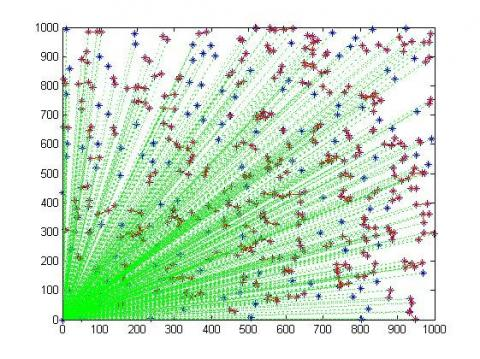(a)

## 2b.png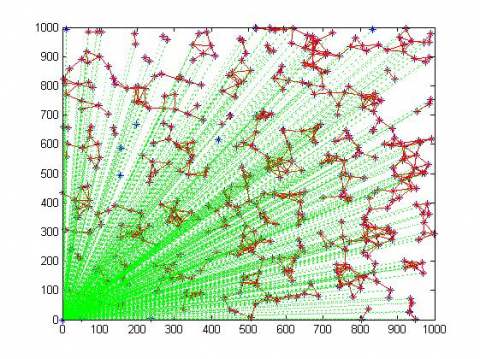(b)

## 2c.png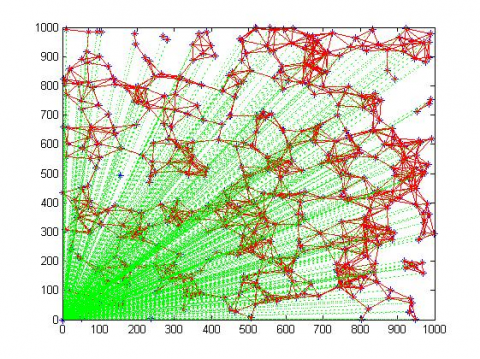(c)

Figure 2. (a) Starting topology of the urban energy network for a d = 30 m; (b) Starting topology of the urban energy network for d = 50 m; (c) Starting topology of the urban energy network for d = 70 m

## 3.png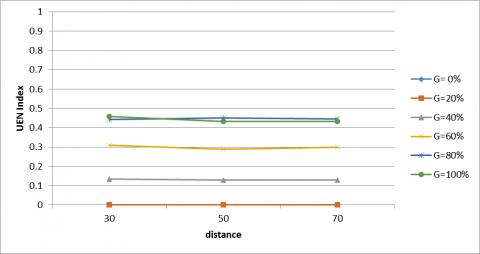Figure 3. Urban energy network index by varying the distance of connection for the different percentages of energy generation

The results are obtained at fixed energy generation, expressed as a percentage of the total energy demand of the urban energy network. The graph permits to infer different considerations. First, by increasing the distance d of connection keeping constant the percentage of energy generation, no increasing of the urban energy network can be observed. Therefore, the distance d of connection is not a discriminating factor in order to obtain a major exploitation of the urban energy network. This is true, at least, for the selected distances. Secondly, the percentage of energy generation seems to have a considerable effect. In fact, except for the percentage of 0% and 20%, a greater number of connections characterize the urban energy network whenever the percentage of energy generation increases. At rigor, the percentage of 0% of energy generation does never activate the links of the urban energy network, since it returns the energy configuration in correspondence of which all nodes are supplied from the central node, i.e. the traditional energy distribution system. For the percentage of 20%, the links of the urban energy network still remains inactivated, because of the fact that nodes use the produced energy for the satisfaction of their own energy demand. Different are the cases of the percentage greater than the 20%. In fact, already for the 40% of the energy generation and until the 80%, the links of the urban energy network are more frequently exploited. Anyway, a further increase of the energy generation does not significantly influence the exploitation of the urban energy network. This is probably due to the fact that neighborhood is served from the nodes at the maximum possibility.

As an example, the optimized urban energy networks corresponding to the 80% of the energy generation are shown in Figure 4.

## 4a.png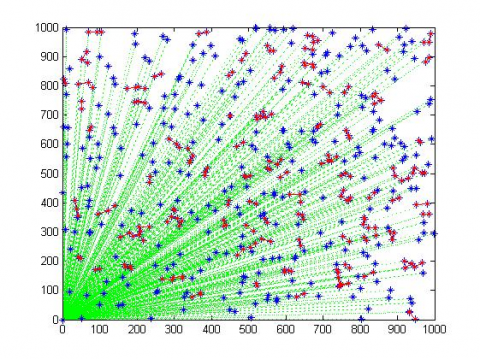(a)

## 4b.png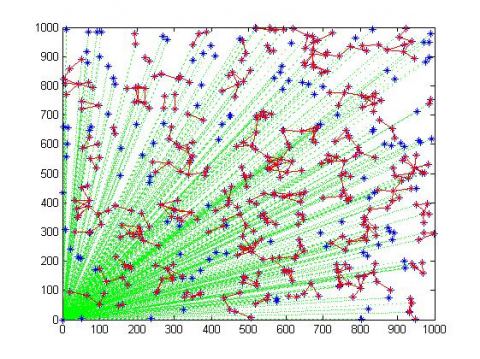(b)

## 4c.png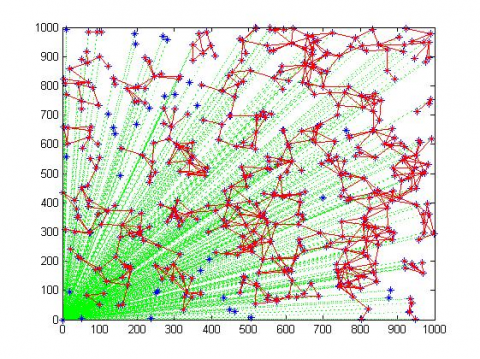(c)

Figure 4. (a) Optimized urban energy network for d = 30 m; (b) Optimized urban energy network for d = 50 m; (c) Optimized urban energy network for d = 70 m

The comparison between Figure 4 and Figure 2 permits to highlight, even at a visual analysis, which the number of connections of the optimized urban energy network, again identified with the red links, decreases in respect of the corresponding starting topology. Regarding the connections with the central node, some of them are still required to ensure the satisfaction of the energy demand of the network.

4. Conclusions

This work introduces a mathematical procedure based on complex networks and its application to a speculative case study for the analysis of the energy distribution within urban areas.

Because Distributed Energy Systems are becoming a key solution for the complex energy requirements of cities, it is essential to elaborate proper energy models for the urban energy planning in cities.

In the developed model, the final users of the energy supply systems have the chance to install DESs for the local production of energy. In this sense, a user is not just a consumer, but may also be identified as a producer. This twofold condition is analyzed with the aim of the complex networks framework. In this direction, users are characterized as nodes with a particular energy demand and, eventually, energy generation. Nodes have the chance to connect according to a vicinity criterion and each connection is represented as a link. The model is then formulated as a mixed integer linear programming model, for which the main objective is to minimize the energy output from the power station, whist encouraging the energy exchanges among private producers.

The model has been tested for a speculative area of 1000 m2 with a homogeneous distribution of users. Particularly, the study pointed out how the percentage of energy generation and the distance of connection influence the exploitation of the links of the urban energy networks. The results emphasizes that the energy generation is a significant factor to take into consideration for ensuring the optimal energy exchanges among users. On the contrary, the distance of connection does not influence the energy behavior of the urban energy network. Nevertheless, it must be say that the range of distance that have been taken into consideration is limited to 70 m, according to the current Italian Regulation for which the energy autonomously produced from privates may be sold only to the immediate neighbors.

Nomenclature
 N number of nodes of the urban energy network Di energy demand of each node i [MWh] Gi energy generation of each node i [MWh] d distance of connection between two nodes of the urban energy network [m] A adjacency matrix of the urban energy network Aopt optimal adjacency matrix of the urban energy network aij elements of the adjacency matrix Si energy surplus that characterizes node i Zij energy flow exchanged between node i and node j UENINDEX urban energy network index linksinvolved number of links that are effectively exploited for the energy distribution on the urban energy network linksvicinity number of links of the starting urban energy network and defined through the vicinity criterion
References

 Directive 2009/28/CE, on the promotion of the use of energy from renewable sources.

 Directive 2010/31/UE, on the energy performance of buildings.

 International Energy Agency, Key World Energy STATISTICS, Edition 2015, available at http://www.iea.org/publications/freepublications/publication/key-world-energy-statistics-2015.html

 A. M. Adil and Y. Ko, “Socio-technical evolution of Decentralized Energy Systems: A critical review and implications for urban planning and policy,” Renewable and Sustainable Energy Reviews, vol. 57, pp. 1025-1037, May 2016.  DOI: http://dx.doi.org/10.1016/j.eser.2015.12.079.

 H. Farzaneh, C. N. H. Doll and J. A. Puppim de Oliveira, “An integrated supply-demand model for the optimization of energy flow in the urban system,” Journal of Cleaner Production, vol. 114, pp. 269-285, May 2015. DOI: http://dx.doi.org/10.1016/j.jclepro.2015.05.098.

 M. La Gennusa, G. Lascari, G. Rizzo, G. Scaccianoce and G. Sorrentino, “A model for predicting the potential diffusion of solar energy systems in complex urban environments,” Energy Policy, vol. 39, pp. 5335-5343, May 2011. DOI: http://dx.doi.org/10.1016/j.enpol.2011.05.031.

 J. Kanters and M. Wall, “A planning process map for solar buildings in urban environments,” Renewable and Sustainable Energy Reviews, vol. 57, pp. 173-185, January 2016. DOI: http://dx.doi.org/10.1016/j.rser.2015.12.073.

 M. Amado and F. Poggi, “Solar urban planning: a parametric approach,” Energy Procedia, vol. 48, pp. 1539-1548, 2014. DOI: http://dx.doi.org/10.1016/j.egypro.2014.02.174.

 A. Semanche, A. Hamidat and A. Benchatti. “Impact study of the solar energy on the energy performances of the rural housing in Algeria,” International Journal of Heat and Technology, vol. 33, no. 4, pp. 229-236, 2015. DOI: http://dx.doi.org/10.18280/ijht.330431.

 F. Ascione, R. F. De Masi, F. De Rossi, R. Fistola, M. Sasso and G. P. Vanoli, “Analysis and diagnosis of the energy performance of buildings and districts: Methodology, validation and development of Urban Energy Maps,” Cities, vol. 35, pp. 270-283, May 2013. DOI: http://dx.doi.org/10.1016/j.cities.2013.04.012.

 K. Orehouning, R. Evins and V. Dorer, “Integration of decentralized energy systems in neighborhoods using the energy hub approach,” Applied Energy, vol. 154. Pp- 277-289, May 2015. DOI: http://dx.doi.org/10.10167j.apenergy.2015.04.114.

 H. Ren and W. Gao, “A MILP model for integrated plan and evaluation of distributed energy systems,” Applied Energy, vol. 87, pp. 1001-1014, October 2009. DOI: http://dx.doi.org/10.1016/j.apenergy.2009.09.023.

 E. D. Mehleri, H. Sarimveis, N. C. Markatos and L. G. Papageorgiou, “A mathematical programming approach for optimal design of distributed energy systems at neighborhood level,” Energy, vol. 44, pp. 96-104, March 2012. DOI: http://dx.doi.org/10.10167j.energy.2012.02.009.

 A. Omu, R. Choudhary and A. Boies, “Distributed energy resource system optimization using mixed integer linear programming,” Energy Policy, vol. 61, pp. 249-266, July 2013. DOI: http://dx.doi.org/10.1016/j.enpol.2013.05.009.

 A. Fichera, R. Volpe and M. Frasca, “Assessment of the energy distribution in urban areas by using the framework of complex network theory,” International Journal of Heat and Technology, vol. 34, special issue 2, pp. S430-S434, October 2016. DOI: http://doi.org/10.18280/ijht.34S234.

 M. E. J. Newman, Networks: An introduction. Oxford, UK: Oxford University Press, 2010.

 [M. Batty, “Editorial. Cities as flows, cities of flows,” Environment and Planning B: Planning and Design, vol. 38, pp. 195-196, 2011. DOI: http://dx.doi.org/10.1068/b3802ed.

 R. Volpe, M. Frasca, A. Fichera and L. Fortuna, “The role of autonomous energy production systems in urban energy networks,” Journal of Complex Networks September 2016, pp. 1-12. DOI: http://dx.doi.org/10.1093/comnet/cnw023.

 Delibera del 7 agosto 2014 426/2014/R/EEL, available at http://www.gse.it/it/Qualifiche%20e%20certificati/Qualifiche_SEU_SEESEU/Pagine/default.aspxx